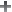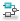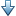## Step Tracking Goal

### Purpose

Make the step response from specified inputs to specified outputs closely match a target response, when using Control System Tuner.

### Description

Step Tracking Goal constrains the step response between the specified signal locations to match the step response of a stable reference system. The constraint is satisfied when the relative difference between the tuned and target responses falls within the tolerance you specify. You can use this goal to constrain a SISO or MIMO response of your control system.

You can specify the reference system for the target step response in terms of first-order system characteristics (time constant) or second-order system characteristics (natural frequency and percent overshoot). Alternatively, you can specify a custom reference system as a numeric LTI model.#### Creation

In the Tuning tab of Control System Tuner, select New Goal > Tracking of step commands to create a Step Tracking Goal.

#### Command-Line Equivalent

When tuning control systems at the command line, use `TuningGoal.StepTracking` to specify a step response goal.

### Step Response Selection

Use this section of the dialog box to specify input, output, and loop-opening locations for evaluating the tuning goal.

• Specify step-response inputs

Select one or more signal locations in your model at which to apply the step input. To constrain a SISO response, select a single-valued input signal. For example, to constrain the step response from a location named `'u'` to a location named `'y'`, clickAdd signal to list and select `'u'`. To constrain a MIMO response, select multiple signals or a vector-valued signal.

• Specify step-response outputs

Select one or more signal locations in your model at which to measure the response to the step input. To constrain a SISO response, select a single-valued output signal. For example, to constrain the step response from a location named `'u'` to a location named `'y'`, clickAdd signal to list and select `'y'`. To constrain a MIMO response, select multiple signals or a vector-valued signal. For MIMO systems, the number of outputs must equal the number of inputs.

• Compute step response with the following loops open

Select one or more signal locations in your model at which to open a feedback loop for the purpose of evaluating this tuning goal. The tuning goal is evaluated against the open-loop configuration created by opening feedback loops at the locations you identify. For example, to evaluate the tuning goal with an opening at a location named `'x'`, clickAdd signal to list and select `'x'`.

Tip

To highlight any selected signal in the Simulink® model, click. To remove a signal from the input or output list, click. When you have selected multiple signals, you can reorder them usingand. For more information on how to specify signal locations for a tuning goal, see Specify Goals for Interactive Tuning.

### Desired Response

Use this section of the dialog box to specify the shape of the desired step response.

• First-order characteristics

Specify the desired step response (the reference model Href) as a first-order response with time constant τ:

`${H}_{ref}=\frac{1/\tau }{s+1/\tau }.$`

Enter the desired value for τ in the Time Constant text box. Specify τ in the time units of your model.

• Second-order characteristics

Specify the desired step response as a second-order response with time constant τ, and natural frequency 1/τ.

Enter the desired value for τ in the Time Constant text box. Specify τ in the time units of your model.

Enter the target overshoot percentage in the Overshoot text box.

The second-order reference system has the form:

`${H}_{ref}=\frac{{\left(1/\tau \right)}^{2}}{{s}^{2}+2\left(\zeta /\tau \right)s+{\left(1/\tau \right)}^{2}}.$`

The damping constant ζ is related to the overshoot percentage by ζ = `cos(atan2(pi,-log(overshoot/100)))`.

• Custom reference model

Specify the reference system for the desired step response as a dynamic system model, such as a `tf`, `zpk`, or `ss` model.

Enter the name of the reference model in the MATLAB® workspace in the LTI model to match text field. Alternatively, enter a command to create a suitable reference model, such as ```tf(1,[1 1.414 1])```.

The reference model must be stable and must have DC gain of 1 (zero steady-state error). The model can be continuous or discrete. If the model is discrete, it can include time delays which are treated as poles at `z` = 0.

The reference model can be MIMO, provided that it is square and that its DC singular value (`sigma`) is 1. Then number of inputs and outputs of the reference model must match the dimensions of the inputs and outputs specified for the step response goal.

For best results, the reference model should also include intrinsic system characteristics such as non-minimum-phase zeros (undershoot).

If your selected inputs and outputs define a MIMO system and you apply a SISO reference system, the software attempts to match the diagonal channels of the MIMO system. In that case, cross-couplings tend to be minimized.

### Options

Use this section of the dialog box to specify additional characteristics of the step response goal.

• Keep % mismatch below

Specify the relative matching error between the actual (tuned) step response and the target step response. Increase this value to loosen the matching tolerance. The relative matching error, erel, is defined as:

`${e}_{rel}=\frac{{‖y\left(t\right)-{y}_{ref}\left(t\right)‖}_{2}}{{‖1-{y}_{ref}\left(t\right)‖}_{2}}.$`

y(t) – yref(t) is the response mismatch, and 1 – yref(t) is the step-tracking error of the target model. ${‖\text{\hspace{0.17em}}\cdot \text{\hspace{0.17em}}‖}_{2}$ denotes the signal energy (2-norm).

For a MIMO tuning goal, when the choice of units results in a mix of small and large signals in different channels of the response, this option allows you to specify the relative amplitude of each entry in the vector-valued step input. This information is used to scale the off-diagonal terms in the transfer function from reference to tracking error. This scaling ensures that cross-couplings are measured relative to the amplitude of each reference signal.

For example, suppose that tuning goal is that outputs `'y1' and 'y2'` track reference signals `'r1'and 'r2'`. Suppose further that you require the outputs to track the references with less than 10% cross-coupling. If `r1` and `r2` have comparable amplitudes, then it is sufficient to keep the gains from `r1` to `y2` and `r2` and `y1` below 0.1. However, if `r1` is 100 times larger than `r2`, the gain from `r1` to `y2` must be less than 0.001 to ensure that `r1` changes `y2` by less than 10% of the `r2` target. To ensure this result, set Adjust for step amplitude to `Yes`. Then, enter `[100,1]` in the Amplitudes of step commands text box. Doing so tells Control System Tuner to take into account that the first reference signal is 100 times greater than the second reference signal.

The default value, `No` , means no scaling is applied.

• Apply goal to

Use this option when tuning multiple models at once, such as an array of models obtained by linearizing a Simulink model at different operating points or block-parameter values. By default, active tuning goals are enforced for all models. To enforce a tuning requirement for a subset of models in an array, select Only Models. Then, enter the array indices of the models for which the goal is enforced. For example, suppose you want to apply the tuning goal to the second, third, and fourth models in a model array. To restrict enforcement of the requirement, enter `2:4` in the Only Models text box.

### Algorithms

When you tune a control system, the software converts each tuning goal into a normalized scalar value f(x). Here, x is the vector of free (tunable) parameters in the control system. The software then adjusts the parameter values to minimize f(x) or to drive f(x) below 1 if the tuning goal is a hard constraint.

For Step Response Goal, f(x) is given by:

`$f\left(x\right)=\frac{{‖\frac{1}{s}\left(T\left(s,x\right)-{H}_{ref}\left(s\right)\right)‖}_{2}}{{e}_{rel}{‖\frac{1}{s}\left({H}_{ref}\left(s\right)-I\right)‖}_{2}}.$`

T(s,x) is the closed-loop transfer function between the specified inputs and outputs, evaluated with parameter values x. Href(s) is the reference model. erel is the relative error (see Options). ${‖\text{\hspace{0.17em}}\cdot \text{\hspace{0.17em}}‖}_{2}$ denotes the H2 norm (see `norm`).

This tuning goal also imposes an implicit stability constraint on the closed-loop transfer function between the specified inputs to outputs, evaluated with loops opened at the specified loop-opening locations. The dynamics affected by this implicit constraint are the stabilized dynamics for this tuning goal. The Minimum decay rate and Maximum natural frequency tuning options control the lower and upper bounds on these implicitly constrained dynamics. If the optimization fails to meet the default bounds, or if the default bounds conflict with other requirements, on the Tuning tab, use Tuning Options to change the defaults.